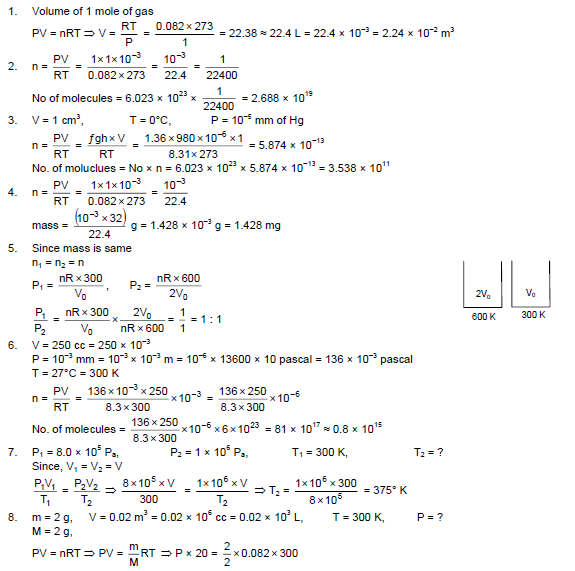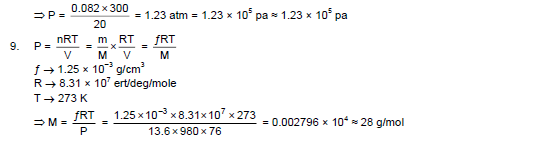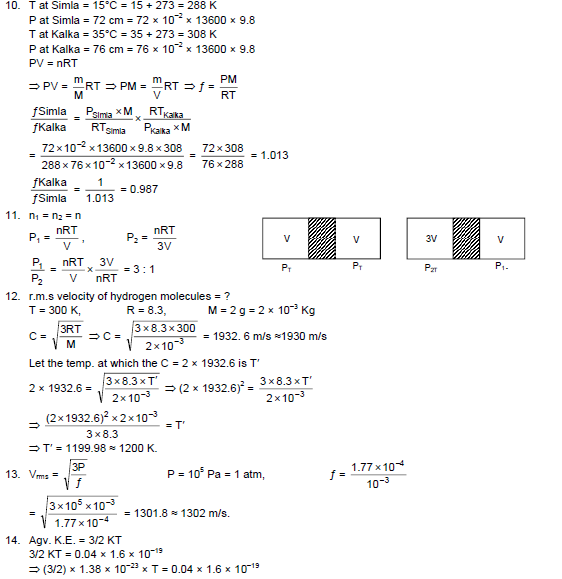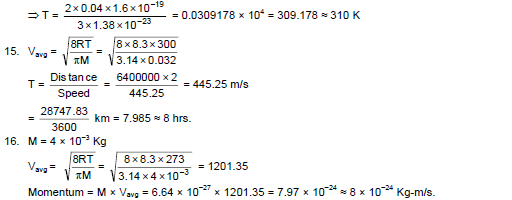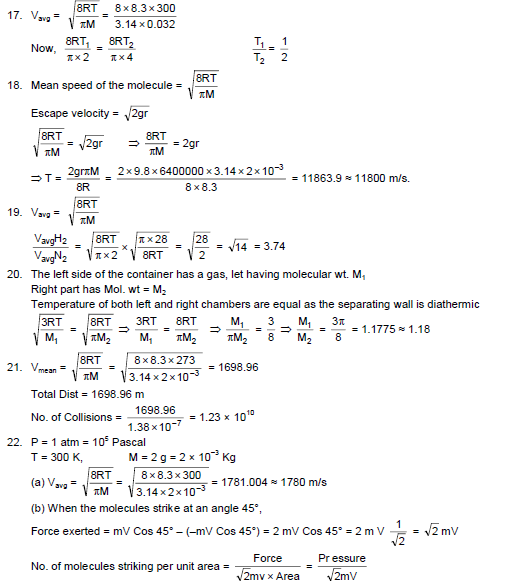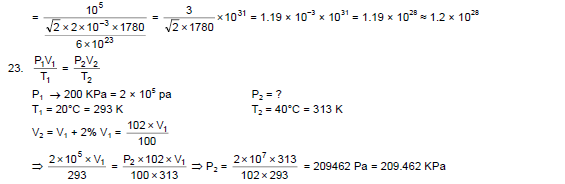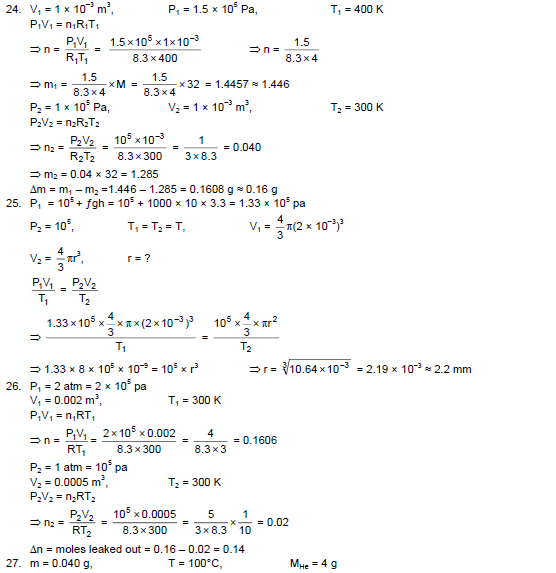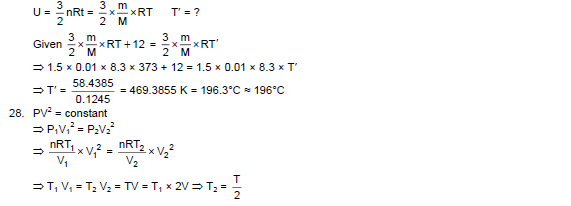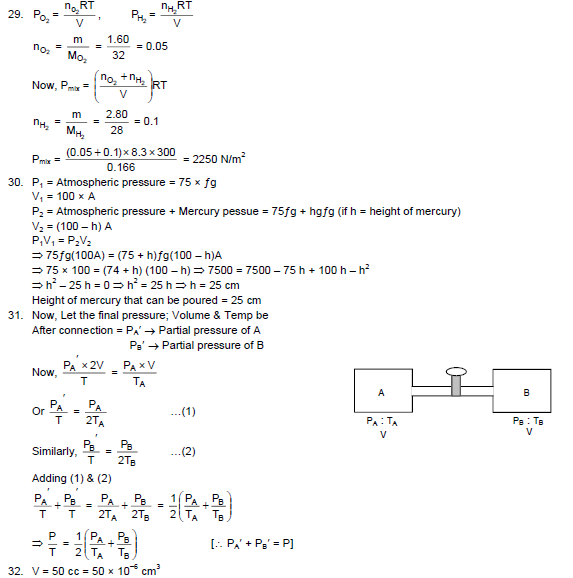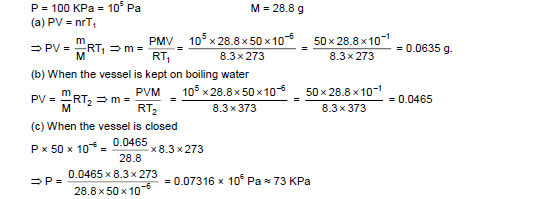# HC Verma Solutions Vol 2 Chapter 2 Kinetic Theory Of Gases

HC Verma Book Vol 2 Chapter 2 Kinetic Theory of Gases is authored by Harish Chandra Verma a retired professor from IIT Kanpur. Kinetic Theory of Gases is one of the important concepts in physics which students need to master and be familiar with. This theory was established in the 19th century by Austrian physicist Ludwig Boltzmann and British scientist James C Maxwell. Besides, in this chapter of the HC Verma book students will study the microscopic behaviour of molecules and their interactions. They will further learn about the macroscopic properties of gas like thermal conductivity, volume, viscosity,  temperature, humidity, vapour, evaporation and pressure in accordance with the kinetic theory.

Additionally, the chapter contains exercise problems that are based on Boyle’s law, Charles’s law, Gay Lussac’s law, Avogadro’s law, Perfect gas equation, Van der Waals’ gas equation, the Kinetic energy of a gas, Degree of freedom, Brownian motion, etc. Practising these solutions will help students improve their problem-solving approach to complex questions on the Kinetic Theory of Gases. To aid students in their preparation, we are providing free HC Verma solutions for this chapter. The solutions contain detailed and accurate answers to all the exercise given in chapter 2. Students can refer the solutions and learn the right way to solve questions.

## Key Topics Related To Kinetic Theory Of Gases

• Assumptions Of Kinetic Theory of Gases
• Kinetic Interpretation of Temperature
• rms Speed
• Determination of Relative Humidity
• Thermodynamic State
• Maxwell’s Speed Distribution Law

## Important Questions In Chapter 2

1. What do you understand when we say that the temperature of all the molecules in a sample of a gas is the same. Give your thoughts on the statement.

2. When we use a pressure cooker to prepare food it is usually faster. Explain.

3. What will the energy of a given sample of an ideal gas depend on?

(a) Pressure (b) Volume (c) Density (d) Temperature

4. Can you state a condition where a normal gas behaves more like an ideal gas?

(a) At high pressure and low temperature (b) At low pressure and high temperature (c) At low pressure and low temperature (d) At high pressure and high temperature

5. Let’s take the rms speed of oxygen molecules in a gas to be v. Now, if the temperature is doubled where the oxygen molecules dissociate into oxygen atoms, what will happen to the rms speed? Choose the right option from the following.

(a) v (b) v2 (c) 2v (d) 4v

## HC Verma Solutions Vol 2 Kinetic Theory of Gases Chapter 2